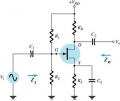Determining signal (internal) resistance and bypass/coupling capacitance values for JFET circuit

bdn1790

Joined Dec 31, 2018
13
A basic common-source potential divider JFET circuit is given with existing values, and with me calculating them with the help of another user in this forum:$$V_{DD} = 20V$$ (Power Supply)
$$I_{DSS} = 10mA$$
$$I_{DQ} = 3mA$$
$$V_G = 1.728V$$
$$V_{GSQ} = -1.357V$$
$$V_{GS(OFF)} = -3V$$
$$V_{DS} = 7V$$
$$g_m = 3.51mS$$
$$R_S = 1028\Omega$$
$$R_D = 3305\Omega$$
$$R_1 = 1585450\Omega$$
$$R_2 = 150k\Omega$$
$$R_L = 10k\Omega$$
$$r_d = \infty$$ (negligible)My objective now is to find the bypass/coupling capacitance values (C1/Cs/C2) and the signal (internal) resistance using a low-frequency small signal analysis so that I can find the cutoff frequencies:$$f_{L_{C_{i}}}, f_{L_{C_{o}}}, f_{L_{C_{s}}}$$

$$f_{L_{C_{i}}}$$ = Lower cut-off frequency at capacitor Ci = $$\frac{1}{2\pi(R_{sig}+(R_1||R_2))C_i}$$

$$f_{L_{C_{o}}}$$ = Lower cut-off frequency at capacitor Co = $$\frac{1}{2\pi(R_D+R_L)C_o}$$

$$f_{L_{C_{s}}}$$ = Lower cut-off frequency at capacitor Cs = $$\frac{1}{2\pi(R_{eq})C_s}$$, where $$R_{eq}$$ = $$R_S || \frac{1}{g_m}$$

I can find the equivalent resistance from the bypass capacitor Cs at the source resistance Rs using the above formula, but then what to do next? I do not have the values of Rsig (signal resistance) and the capacitance values. The solutions I have tried are:

1. I attempted to use this online resource in finding Rsig. Though, my assignment question did not provide the value of Vsig so I am unable to use the equations provided in that resource.

2. I am unable to use the internal/stray capacitance that become available at high frequency, because I am supposed to solve this at low frequency.

3. Although I can find the voltage gain at midrange frequency, I fail to see how it can help me in finding the aforementioned values unless I am missing out on something.

Pointing me in the right direction would be very helpful. Thanks!

Last edited:

dl324

Joined Mar 30, 2015
13,846
Schematic referenced:Is this school work?

bdn1790

Joined Dec 31, 2018
13

dl324

Joined Mar 30, 2015
13,846
Yes, university assignment.
You need to post your work so members can see where you're having difficulty and can offer guidance.

Jony130

Joined Feb 17, 2009
5,292

(negligible)

OMG, electronic is not the math classes. Such precision in the calculation is pointless. It is impossible to buy 1028.255451Ω ohms resistor.
And most resistors we are using will have 5% tolerances.

Did you ever heard about E24 series?

I can find the cutoff frequencies
.
And F_LG and others are equal to what?

Next, you need to find the equivalent resistance "seen" from the capacitor's terminal (from the capacitors point of view).

bdn1790

Joined Dec 31, 2018
13
And F_LG and others are equal to what?

Next, you need to find the equivalent resistance "seen" from the capacitor's terminal (from the capacitors point of view).
I apologize for giving the exact resistance values. I have updated the thread with more information, hopefully that can better help you understand what my problem is. I have also updated the thread with the formula for the equivalent resistance. I can also find the value of the equivalent resistance. But what to do from there? Do I have to analyze the small-signal circuit using KVL at the capacitance, or?

Jony130

Joined Feb 17, 2009
5,292
If in your technical requirement do not specify the lower cutoff frequency. You can choose it yourself between 10Hz...500Hz.
Or simply write that the capacitor reactance Xc should be equal or lower than the equivalent resistance seen by the capacitor at the lowest frequency you care about. Xc ≤ Req. As for the Rsig if not specified simply assume Rsig = 0Ω.